# Excel WBS and Gantt Chart - XLGantt How to #14 – Workload calculation

Let's take a look at how to calculate the workload when scheduling a project.

As shown below, if the start date of the “Design database” task is July 22 and the finish date is July 31, the working days(excluding nonworking days) are 8.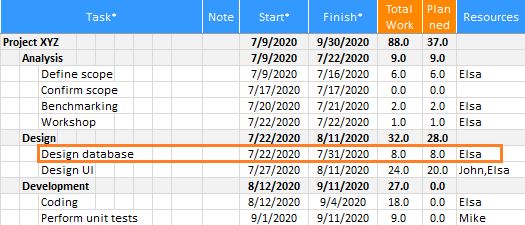※ Refer to the following link for setting nonworking days and calculating the workload.

XLGantt(Excel Gantt) How to #11 – Calendar

If only one person is assigned half a day or several people are assigned to the “Design database” task, the workload should be calculated as follows.

• If only 50% (only half a day) is allocated, the workload should be calculated as 8 days * 50% = 4 days.
• If 2 people are assigned, the workload should be calculated as 8 days * 2 people = 16 days.

For the above case, XLGantt provides the following two methods of calculating the workload.

• Calculated by resource allocation ratio (provided from version 4.0)
• Calculated by weight## 1. Calculated by resource allocation ratio

"Calculated by resource allocation ratio" is a feature added from version 4.0.

If you enter “Elsa, Mike” or “Elas[50%], Mike" in the "Resources" column of the Schedule sheet, the workload is calculated according to the percentage of the assigned personnel.
You can check the workload assigned to each person in the "Progresses by Resources" and "Workloads" features(You can use the feature from the ribbon menu XLGantt tab> Analysis group).

### 1) When one person is assigned to one task

If nothing is entered in the "Resources" column or if only one name of the assigned personnel is entered, it is assumed that one person is assigned.

If nothing is entered in the "Resources" column, it is assumed that one person is assigned and the total workload is calculated as 8 days.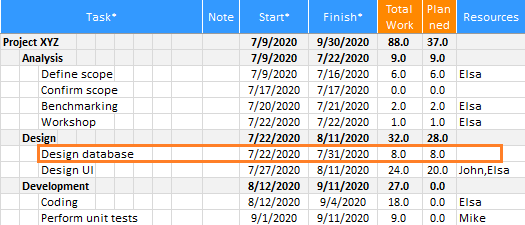if only one name of the assigned personnel is entered in the resources column, it is assumed that one person is assigned and the total workload is calculated as 8 days.※ The “planned work” displayed on the right side of the "total work" is the workload to be carried out until today. The basic calculation method is the same as the "total work", except that it is calculated by the working days until today.

### 2) When a part of the resource is allocated to one task

If you enter “Elsa [50%]” in the "Resources" column, only half will be calculated.

Total workload = number of working days excluding nonworking days  * Number of entered people (Elsa [50%])
4 days = 8 days * 50% (0.5 Elsa)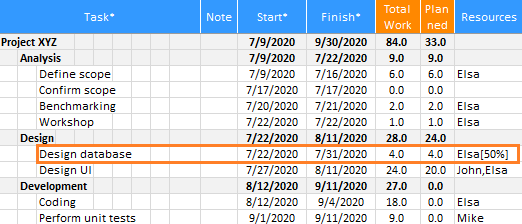### 3) When multiple people are assigned to a single task

If you enter multiple names in the resources column, the workload is calculated as follows.

Total workload = number of working days excluding nonworking days * Number of people entered (Elsa, Mike)
16 days = 8 days * 2 people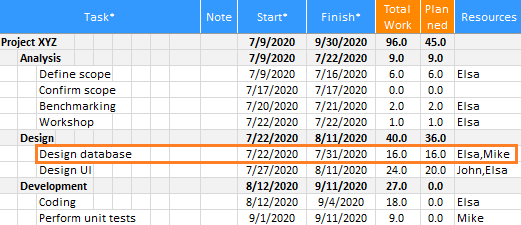The result is the same as above even if you enter "Elsa[100%], Mike[100%]" as follows.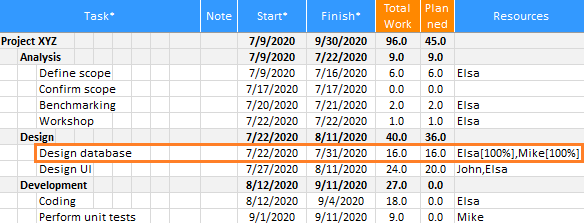It can be calculated even if several people are assigned as follows or only a part of a specific person is assigned.

Total workload = Number of working days excluding nonworking days * Number of people entered (Elsa[50%], Mike)
12 days = (8 days * 50% (0.5 Elsa)) + (8 days * 1 Mike)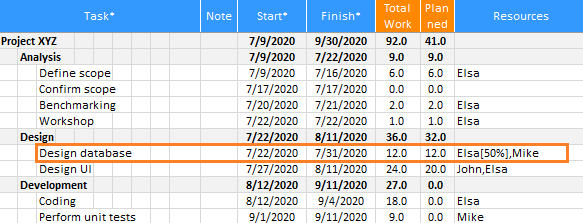If you click “Progresses by Resources"(ribbon menu> XLGantt tab > Analysis group > Progresses by Resources), you can check the result as follows.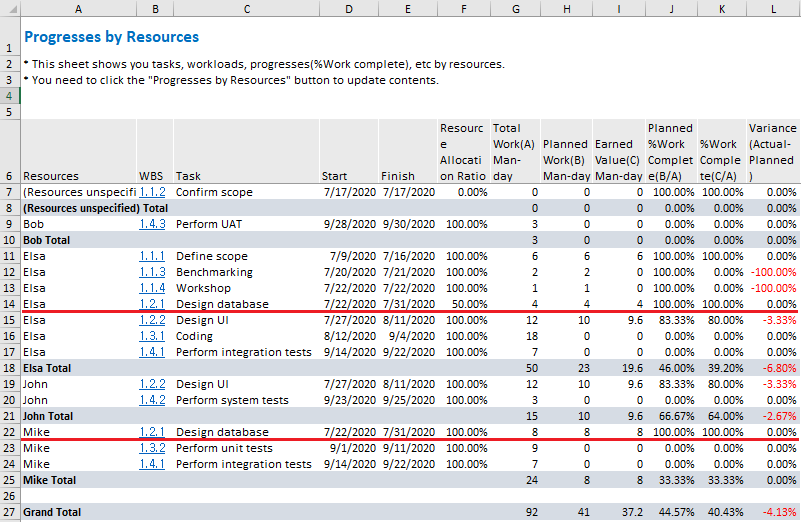### 4) Application

If you enter "Elsa [100%]" in the "Resources" column, one person will be assigned full time (8 hours if a working condition is 8 hours a day), and if you enter "Elsa [200%]", Elsa will be assigned twice.

If you don't know the names of the people assigned to the “Design database” task, but 10 people are assigned, if you enter “Designer”, the workload is calculated as 10 designers are assigned to the task(designer is the same as designer[1000%]).

Total workload = number of working days excluding nonworking days  * Number of entered persons (10 designers)
80 days = 8 days * 10 people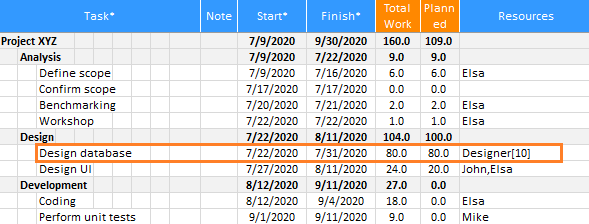## 2. Calculated by weight

The "Calculated by weight" feature was provided before the upgrade to XLGantt version 4.0.

This feature is provided for compatibility with the previous version, and calculation is possible when only part of the person is allocated or when multiple people are allocated, but it is not recommended because there is a problem that the allocation ratio of a specific person cannot be specified.

### 1) When one person is assigned to one task

If nothing is entered in the "Weight" column or 1 is entered, the total workload is calculated as 8 days as one person is assigned.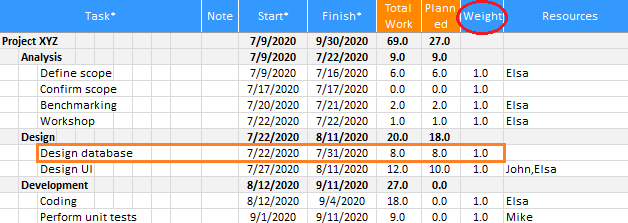### 2) When a part of the resource is allocated to one task

If you enter “0.5” in the "Weight" column, only half will be calculated.

Total workload = number of working days excluding nonworking days * weight
4 days = 8 days * Weight 0.5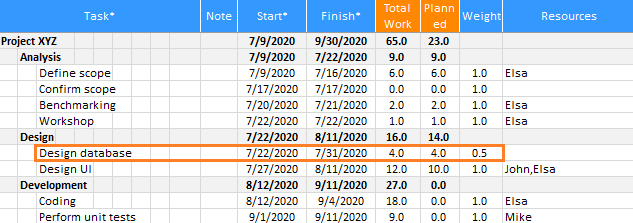### 3) When multiple people are assigned to a single task

If you enter 2 in the "Weight" column, it is calculated that two people are assigned.

Total workload = number of working days excluding nonworking days * weight
16 days = 8 days * Weight 2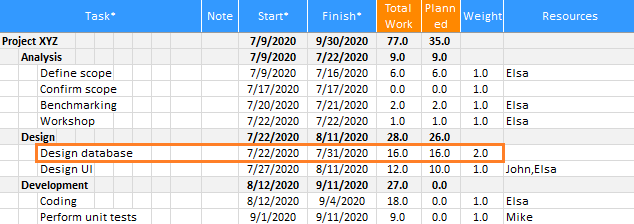The workload is calculated even if the name of the resource is not entered in the "Resources" column.

If 2 people's names are entered, the workload is calculated at a ratio of 100% (weight 2 / 2 people = 100%) for each person.

If 3 people's names are entered with the weight of 2, the workload is calculated at a ratio of 66.7% (weight 2/3 people = 66.7%) for each person, but there is a problem that the allocation ratio of a specific person cannot be adjusted.

If you select the "Calculated by resource allocation ratio" option, you can adjust the allocation ratio of a specific person.

[ XLGantt(Excel Gantt) How to ]
Scroll to Top